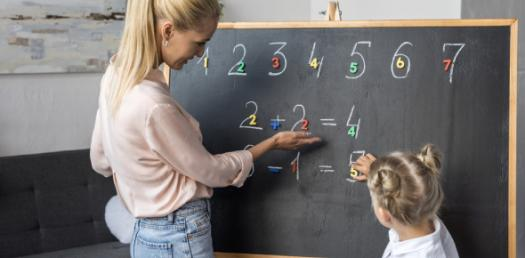# Addition Quiz: Take This Ultimate Math Trivia Test!

48 Questions | Total Attempts: 1576SettingsThis is the ultimate math trivia to see how good your skills are. Our quiz looks to test your skills necessary to solve these problems. These questions will evaluate your math level, so be sure to take our quiz and see how easy it is to solve these concepts. All the best and check out other quizzes like it!

Questions and Answers
• 1.
2+4=
• 2.
4+2=
• 3.
2+6=
• 4.
7+0=
• 5.
3+4=
• 6.
4+3=
• 7.
6+2=
• 8.
5+3=
• 9.
0+7=
• 10.
2+6=
• 11.
2+5=
• 12.
3+3=
• 13.
4+1=
• 14.
3+4=
• 15.
6+0=
• 16.
5+2=
• 17.
5+3=
• 18.
3+2=
• 19.
4+3=
• 20.
1+7=
• 21.
3+4=
• 22.
6+2=
• 23.
4+4=
• 24.
4+2=
• 25.
7+1=
Related Topics